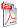Issue archive

https://doi.org/10.15255/KUI.2020.002
Published: Kem. Ind. 69 (11-12) (2020) 595–602
Paper reference number: KUI-02/2020
Paper type: Original scientific paper
Download paper:PDFPrediction of the Bicarbonate Amount in Drinking Water in the Region of Médéa Using Artificial Neural Network Modelling

H. Tahraoui, A. E. Belhadj and A. E. Hamitouche

##### Abstract

The region of Médéa (Algeria) located in an agricultural site requires a large amount of drinking water. For this purpose, the water analyses in question are imperative. To examine the evolution of the drinking water quality in this region, firstly, an experimental protocol was done in order to obtain a dataset by taking into account several physicochemical parameters. Secondly, the obtained data set was divided into two parts to form the artificial neural network, where 70 % of the data set was used for training, and the remaining 30 % was also divided into two equal parts: one for testing and the other for validation of the model. The intelligent model obtained was evaluated as a function of the correlation coefficient nearest to 1 and lowest mean square error (RMSE). A set of 84 data points were used in this study. Eighteen parameters in the input layer, five neurons in the hidden layer, and one parameter in the output layer were used for the ANN modelling. Levenberg Marquardt learning (LM) algorithm, logarithmic sigmoid, and linear transfer function were used, respectively, for the hidden and the output layers. The results obtained during the present study showed a correlation coefficient of R = 0.99276 with root mean square error RMSE = 11.52613 mg dm–3. These results show that obtained ANN model gave far better and more significant results. It is obviously more accurate since its relative error is small with a correlation coefficient close to unity. Finally, it can be concluded that obtained model can effectively predict the rate of soluble bicarbonate in drinking water in the Médéa region.

##### Keywords

drinking water, physicochemical parameters, bicarbonate, modelling